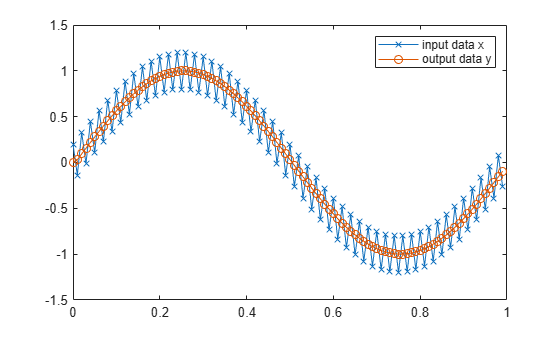# subsasgn

Subscripted assignment

## Syntax

``A = subsasgn(A,S,B)``

## Description

example

````A = subsasgn(A,S,B)` is called for the syntax `A(i) = B`, `A{i} = B`, or `A.i = B` when `A` is an object.MATLAB® uses the built-in `subsasgn` function to interpret indexed assignment statements: `A(i) = B` assigns the values of `B` into the elements of `A` specified by the subscript vector `i`. `B` must have the same number of elements as `i` or be a scalar value.`A(i,j) = B` assigns the values of `B` into the elements of the rectangular submatrix of `A` specified by the subscript vectors `i` and `j`. `B` must have `length(i)` rows and `length(j)` columns.A colon used as a subscript, as in `A(i,:) = B` or `A(:,i) = B`, indicates the entire column or row.For multidimensional arrays, `A(i,j,k,…) = B` assigns `B` to the specified elements of `A`. `B` must be `length(i)`-by-`length(j)`-by-`length(k)`-… or be shiftable to that size by adding or removing singleton dimensions. TipYou can use fixed-point assignment, for example, `A(:) = B`, to cast a value with one numeric type into another numeric type. This subscripted assignment statement assigns the value of `B` into `A` while keeping the numeric type of `A`. Subscripted assignment works the same way for integer data types. NoteYou must call `subsasgn` with an output argument. `subsasgn` does not modify the object used in the indexing operation (the first argument). You must assign the output to obtain a modified object. ```

## Examples

collapse all

For `fi` objects `a` and `b`, there is a difference between

`a = b`

and

`a(:) = b`.

In the first case, `a = b` replaces `a` with `b` while `a` assumes the value, numeric type, and `fimath` object associated with `b`. In the second case, `a(:) = b` assigns the value of `b` into `a` while keeping the numeric type of `a`. You can use this to cast a value with one `numerictype` object into another `numerictype` object.

For example, cast a 16-bit number into an 8-bit number.

`a = fi(0, 1, 8, 7)`
```a = 0 DataTypeMode: Fixed-point: binary point scaling Signedness: Signed WordLength: 8 FractionLength: 7 ```
`b = fi(pi/4, 1, 16, 15)`
```b = 0.7854 DataTypeMode: Fixed-point: binary point scaling Signedness: Signed WordLength: 16 FractionLength: 15 ```
`a(:) = b`
```a = 0.7891 DataTypeMode: Fixed-point: binary point scaling Signedness: Signed WordLength: 8 FractionLength: 7 ```

Define the variable `acc` to emulate a 40-bit accumulator of a DSP. The products and sums in this example are assigned into the accumulator using the syntax `acc(1)=...`. Assigning values into the accumulator is like storing a value in a register. To begin, turn on the logging mode and define the variables. In this example, `n` is the number of points in the input data `x` and output data `y`, and `t` represents time. The remaining variables are all defined as `fi` objects. The input data `x` is a high-frequency sinusoid added to a low-frequency sinusoid.

```fipref('LoggingMode', 'on'); n = 100; t = (0:n-1)/n; x = fi(sin(2*pi*t) + 0.2*cos(2*pi*50*t)); b = fi([.5 .5]); y = zeros(size(x),'like',x); acc = fi(0.0, true, 40, 30);```

The following loop takes a running average of the input `x` using the coefficients in `b` . Notice that `acc` is assigned into `acc(1)=...` versus using `acc=...`, which would overwrite and change the data type of `acc` .

```for k = 2:n acc(1) = b(1)*x(k); acc(1) = acc + b(2)*x(k-1); y(k) = acc; end```

By averaging every other sample, the loop shown above passes the low-frequency sinusoid through and attenuates the high-frequency sinusoid.

```plot(t,x,'x-',t,y,'o-') legend('input data x','output data y')```The log report shows the minimum and maximum logged values and ranges of the variables used. Because `acc` is assigned into rather than overwritten, these logs reflect the accumulated minimum and maximum values.

`logreport(x, y, b, acc)`
``` minlog maxlog lowerbound upperbound noverflows nunderflows x -1.200012 1.197998 -2 1.999939 0 0 y -0.9990234 0.9990234 -2 1.999939 0 0 b 0.5 0.5 -1 0.9999695 0 0 acc -0.9990234 0.9989929 -512 512 0 0 ```

Display `acc` to verify that its data type did not change.

`acc`
```acc = -0.0941 DataTypeMode: Fixed-point: binary point scaling Signedness: Signed WordLength: 40 FractionLength: 30 ```

Reset the `fipref` object to restore its default values.

`reset(fipref)`

## Input Arguments

collapse all

Object used in indexing operation, specified as a scalar, vector, or multidimensional array.

Data Types: `single` | `double` | `int8` | `int16` | `int32` | `int64` | `uint8` | `uint16` | `uint32` | `uint64` | `logical` | `fi`
Complex Number Support: Yes

Type of indexing and subscripts, specified as a structure array. `S` is a structure array with two fields:

• `type` is a character vector or string containing `()`, `{}`, or `.`, specifying the subscript type.

• `subs` is a cell array, character array, or string array containing the actual subscripts.

Example: The syntax `A(1:2,:) = B` calls ```a = subsasgn(A,S,B)``` where `S` is a 1-by-1 structure with `S.type = '()'` and `S.subs = {1:2, ':'}`. A colon used as a script is passed as `':'`.

Data Types: `struct`

Value being assigned, specified as a scalar, vector, or multidimensional array.

Data Types: `single` | `double` | `int8` | `int16` | `int32` | `int64` | `uint8` | `uint16` | `uint32` | `uint64` | `logical` | `fi`
Complex Number Support: Yes

## Output Arguments

collapse all

Result of assignment statement, which is the modified object passed in as the first argument, returned as a scalar, vector, or multidimensional array.

## Version History

Introduced before R2006a